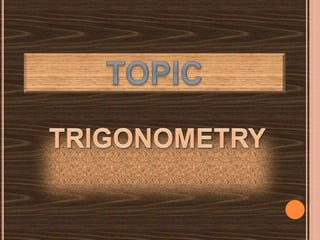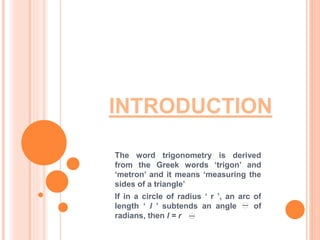Seu SlideShare está sendo baixado. ×
Anúncio
Anúncio
Anúncio
Anúncio
Anúncio
Anúncio
Anúncio
Anúncio
Anúncio
Anúncio
AnúncioCarregando em…3
×

1 de 22 Anúncio

# Mathematics

Power point presentation based on trigonometry, easy to understand, for class XI, good for learning faster and easier, also could be understood by below class XI.

Power point presentation based on trigonometry, easy to understand, for class XI, good for learning faster and easier, also could be understood by below class XI.

Anúncio
Anúncio

### Mathematics

1. 1. By ;- Soumyadeepta Roy Class:- XI ‘B’ Roll no:- 31
2. 2. INTRODUCTION The word trigonometry is derived from the Greek words ‘trigon’ and ‘metron’ and it means ‘measuring the sides of a triangle’ If in a circle of radius ‘ r ’, an arc of length ‘ l ’ subtends an angle of radians, then l = r
3. 3. INTRODUCTION  What about angles greater than 90°? 180°?  The trigonometric functions are defined in terms of a point on a terminal side  r is found by using the Pythagorean Theorem: 22 yxr 
5. 5. THE 6 TRIGONOMETRIC FUNCTIONS OF ANGLE  ARE: sin y r   cos  tan  , sin  0 0 0 csc , sec , cot , r y y r x x x y y         0x 
6. 6. THE TRIGONOMETRIC FUNCTIONS  The trigonometric values do not depend on the selected point – the ratios will be the same:
7. 7. First Quadrant: sin  = + cos  = + tan  = + cosec  = + sec  = + cot  = +
8. 8. y x
9. 9. y x
10. 10. ALL STAR TRIG CLASS  Use the phrase “All Star Trig Class” to remember the signs of the trig functions in different quadrants: AllStar Trig Class All functions are positive Sine is positive Tan is positive Cos is positive
11. 11. The value of any trig function of an angle  is equal to the value of the corresponding trigonometric function of its reference angle, except possibly for the sign. The sign depends on the quadrant that  is in. So, now we know the signs of the trig functions, but what about their values?...
12. 12. REFERENCE ANGLES  The reference angle, α, is the angle between the terminal side and the nearest x-axis:
13. 13. ALL STAR TRIG CLASS  Use the phrase “All Star Trig Class” to remember the signs of the trig functions in different quadrants: AllStar Trig Class All functions are positive Sine is positive Tan is positive Cos is positive
14. 14. TRIGONOMETRIC IDENTITIES  Reciprocal Identities 1 sin csc x x  1 cos sec x x  1 tan cot x x  sin tan cos x x x  cos cot sin x x x  Quotient Identities
15. 15. QUADRATIC ANGLES (TERMINAL SIDE LIES ALONG AN AXIS)
16. 16. THE VALUE OF TRIGONOMETRIC FUNCTIONS FOR SOME COMMON ANGLES. 0˚ ╥/6 ╥/4 ╥/3 ╥/2 ╥ 3╥/2 2 ╥ 0 ½ 1/ 2 3 /2 1 0 -1 0 1 3 /2 1/ 2 ½ 0 -1 0 1 0 1/ 3 1 3 Not defined 0 Not defined 0 sin cos tan
17. 17. TRIGONOMETRIC IDENTITIES 2 2 1sin cosx x  2 2 1 cot cscx x  2 2 1tan secx x   Pythagorean Identities  The fundamental Pythagorean identity:  Divide the first by sin2x :  Divide the first by cos2x :
18. 18. TRIGONOMETRIC IDENTITIES
19. 19. TRIGONOMETRIC IDENTITIES
20. 20. THANK YOU